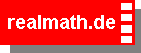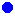On the sheet at the right there's drawn a numbers half-line.

Every fraction is associated with one letter from A to Z .
Pull every single pointto where it belongs.

Watch out:
Some points can be located on top of one another.

When your done, you can get your results checked by
check fractions
and you can get
new fractions
by clicking the button

Can you get 199 points?## Arrange the fractions

 A =
 B =
 C =
 D =
 E =
 F =
 G =
 W =
 Y =
 Z =

© Andreas Meier, Weiden i.d.OPf